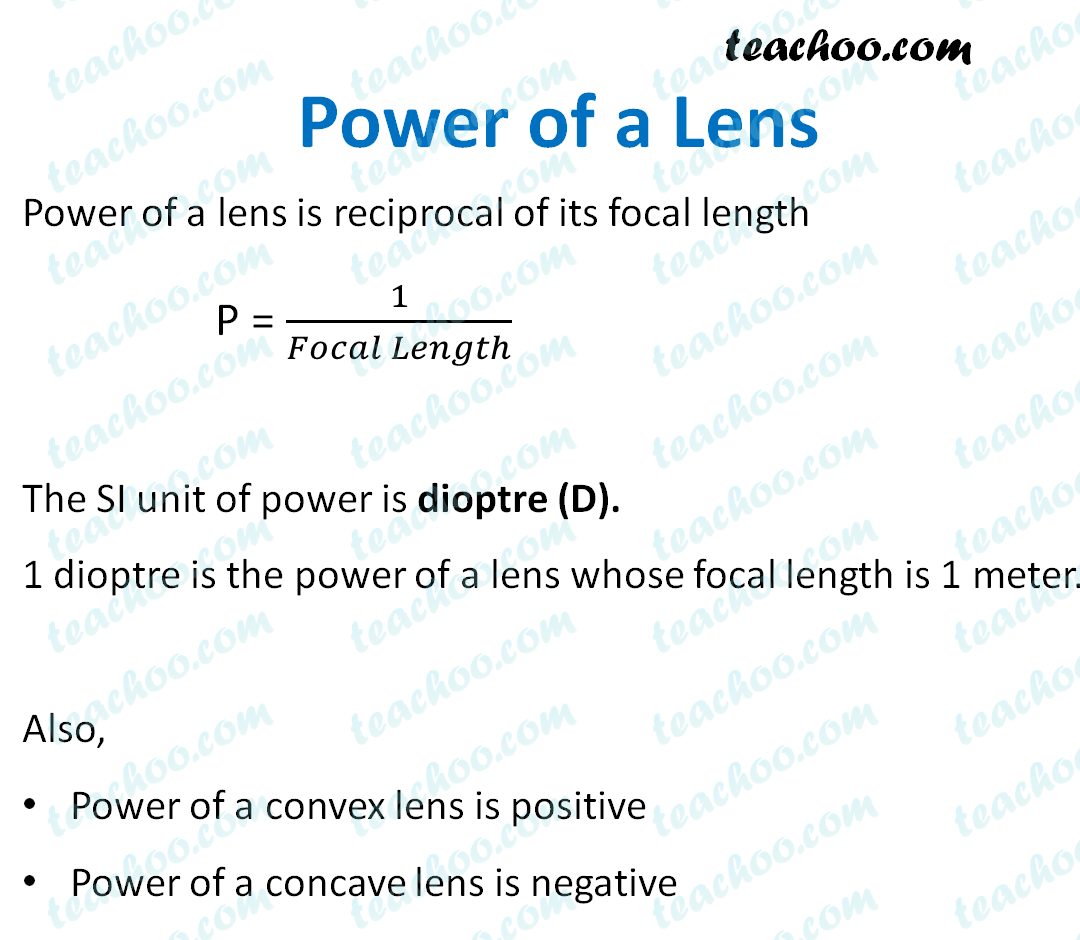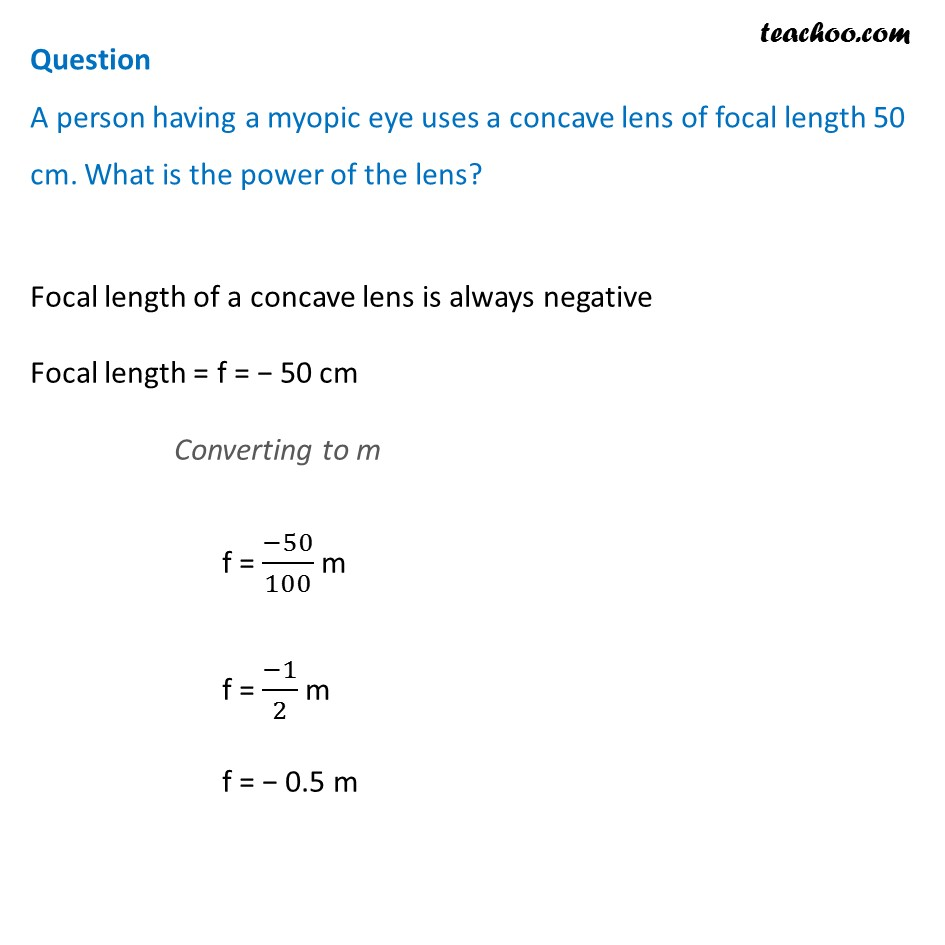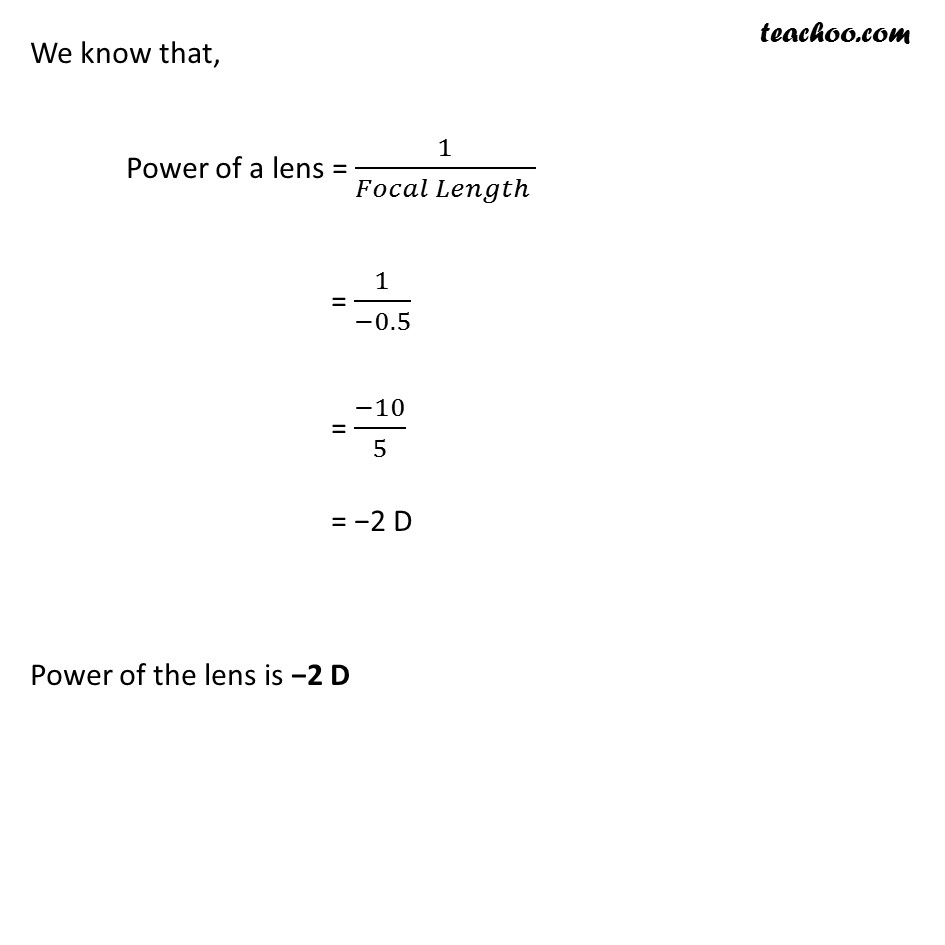Concepts

Class 10
Chapter 10 Class 10 - Light - Reflection and Refraction

It is the Reciprocal of Focal Length taken in m.

Power of lens = 1/Focal Length

P = 1/f

When Focal Length is Measured in meter (m)

Power is Measured in Dioptre (D)

##googletag.cmd.push(function() { googletag.display('div-gpt-ad-1669298377854-0'); });

Let's solve some examples

## Suppose for a concave lens, focal length is 25 cm.What is the power of the lens?

We know that

Concave lens has negative focal length

Therefore

Focal Length = - 25 cm

= - 25/100 m = - 1/4 m

Now,

Power = 1/Focal length

= 1/(-1/4)

= -4 D

Note : -   Focal Length of Convex Lens is Positive. So, Power of Convex lens is also Positive
Focal Length of Concave Lens is Negative. So, Power of Concave lens is also Negative

## A person having a myopic eye uses a concave lens of focal length 50 cm. What is the power of the lens?## Definition of Focal Length

It is the measure of Degree of Convergence and Divergence of a Light Rays

Convex Lens Converges Parallel Rays of Light and Concave Lens Diverges Parallel rays of Light

Power of Lens is Defined as Reciprocal of Focal Length

Lesser the focal Length, More the Power

More the Focal Length, Less the Power

## Power of Combination of Lens

If more than 1 one lens are used together, it is called Combination of Lens

Power of Combination of Lens is

Simple Algebraic sum of all the Lenses

Power of Combination of Lens = Power of Lens 1 + Power of Lens 2

P = P 1 + P 2

## Questions

Question 1 Page 184 - Define 1 dioptre of power of a lens.

Question 2 Page 184 - A convex lens forms a real and inverted image of a needle at a distance of 50 cm from it. Where is the needle placed in front of the convex lens if the image is equal to the size of the object? Also, find the power of the lens

Question 3 Page 184 - Find the power of a concave lens of focal length 2 m.

NCERT Question 16 - Find the focal length of a lens of power – 2.0 D. What type of lens is this?

NCERT Question 17 - A doctor has prescribed a corrective lens of power +1.5 D. Find the focal length of the lens. Is the prescribed lens diverging or converging?

Learn in your speed, with individual attention - Teachoo Maths 1-on-1 Class